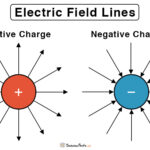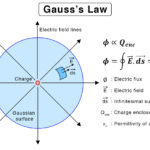Home / Physics / Electric Flux

# Electric Flux

## What is Electric Flux

The number of electric field lines or electric lines of force passing through a given surface area is called electric flux. The area can be in air or vacuum. It can also be inside or on the surface of a solid conductor. The number of lines passing per unit area gives the electric field strength in that region.

## Electric Flux Equation

All flat surfaces have an area associated with them. A regular geometric surface like a circle, rectangle, square, or triangle has a well-defined area. Mathematically, a surface is represented by a vector known as area vector, whose magnitude is the quantified area, and the direction is perpendicular to the surface. Therefore,

A = An

Where,

A: Area vector

A: Magnitude of the area

n: Unit vector perpendicular to the surface

Mathematically, the electric flux is given by the dot product of the electric field vector and area vector.

φ = E.A

Where,

φ: Electric flux

E: Electric field

A: Area vector

If the angle between E and A is θ, then the dot product can be expanded to give the equation for electric flux.

Equation

φ = EA cos θ

Symbol: φ or phi

SI Unit: Volt-meter (V-m) or Newton per meter-squared per Coulomb (Nm2C-1)

CSG Unit: stat Coulomb (statC) or Franklin (Fr) (1 C = 3 x 109 statC)

Dimension: [M L3 T−3 A−1]

When θ = 0˚, cos 0˚ = 1, therefore, φ = EA

A maximum number of electric field lines will pass through the surface when placed perpendicular to the electric field. Also, the electric flux can be negative if the electric field vector and the area vector are in opposite directions.

If E and A arein the same direction, then the flux is positive, otherwise the flux is negative.

Electric Flux Density

The electric flux density is the number of electric field lines passing through a unit area projected perpendicular to the flow direction. Consider the equation,

φ = EA

For unit area, A = 1 and,

φ = E

Therefore, the electric flux density is equal to the magnitude of the electric field.

### Integral Form

The above equation applies to situation where the electric field is uniform and the surface is flat. If the electric field changes in magnitude and/or direction over the entire surface, then it is non-uniform. For non-uniform electric field and curved surface, the electric flux is determined by integration the dot product over the surface. For this purpose, an infinitesimal area is taken. The electric flux us given by the equation,

φ = ∫E.dA

Where dA = dA n is the infinitesimal area vector whose direction is perpendicular to the surface

Further, even if the field is uniform, the surface can be curved. A curve surface can be open or closed, like a sphere, cube, and cylinder. In this case a similar integration is carried out as well.

Electric Flux Through a Sphere

Consider the example of electric field lines passing through a spherical surface, and the lines originate from a point charge. According to Coulomb’s law, the electric field E due to a point charge q at a distance r is given by,

E = q/4πεor2

From the above equation, it is pretty clear that the electric field varies inversely with the square of the distance. Hence, the field is non-uniform. Therefore, to calculate flux passing through a sphere, one has to use the integral form.

Consider an infinitesimal area dA, whose direction is perpendicular to the sphere’s surface. From the symmetry of the problem, the electric field’s E direction is radially outwards. Hence, it is perpendicular to the sphere’s surface as well. Therefore, the angle between E and dA is zero.

E.dA = EdA.

Also, the infinitesimal area of a sphere is given by,

dA = r2sinθdθdψ

Where r, θ, and ψ are the spatial coordinates in a spherical coordinate system.

Therefore, the electric flux enclosed by a sphere is,

φ = ∫ q/(4πεor2) r2sinθdθdψ

or, φ = q/εo

Where,

εo = permittivity of free space (= 8.85 x 10-12 N1m2 C2)

## Applications of Electric Flux

• To calculate the electric field around a charged particle or conductor
• To solve complex problems in electrostatics

Gauss’ law is an essential theorem in physics and one of the concepts it is based on is the electric flux.

## Problems and Solutions

P.1. Find the flux through a spherical surface of radius a = 0.5 m surrounding a charge of 10 pC.

Soln. Given,

q = 10 pC = 10 x 10-12 C = 10-11 C

φ = q/εo = 10-11 C/8.85 x 10-12 N1m2 C2

or, φ = 1.13 Nm2 C-1

P.2. A square surface with a side length of 4.5 mm is located in a uniform electric field with a magnitude of 2200 N/C. The angle between the normal to the surface and the electric field is 30˚. What is the electric flux through the surface?

Soln. Given,

E = 2200 N/C

l = 4.5 mm = 4.5 x 10-3 m

A = l2 = (4.5 x 10-3 m)2 = 2.03 x 10-5 m2

θ = 30˚

Therefore,

φ = EA cos θ = 2200 N/C x 2.03 x 10-5 m2 x cos 30˚

or, φ = 0.039 Nm2C-1

Article was last reviewed on Saturday, September 11, 2021

### Related articlesElectric Field LinesToroid Magnetic FieldGauss’s LawMagnetic Flux